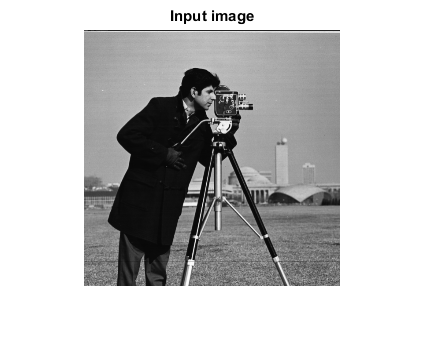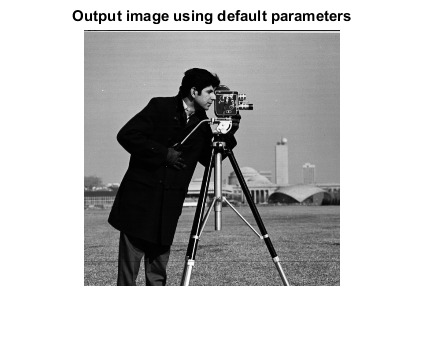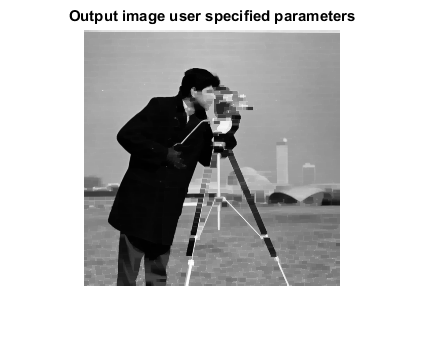# MORPHCLOSE_CVIP

morphclose_cvip() - perform morphological closing of a grayscale or color image.

## SYNTAX

`[outImage, struct_el] = morphclose_cvip(inImage, kType, kSize, kArgs)`

Input Parameters include :

• inImage - 1-band or 3-band image of MxN size.
• kType - Kernel or Structure Elements type. kType = 1 ----> disk kType = 2 ----> square kType = 3 ----> rectangle kType = 4 ----> cross (default: 2)
• kSize - Size of Kernel or Structure Elements. Kernel will a square matrix of K*K, where K = kSize. kSize must be positive odd integer (1,3,5, and so on). (default: 3)
• kArgs - Kernel arguments. The number of arguments vary with the kernel type. Any argument must be positive odd integer (1,3,5, and so on) 'disk' kernel, no arguments, i.e. kArgs = [] 'square' kernel, one argument (width) i.e. kArgs = width (default: kSize) 'rectangle' kernel, two arguments (width & height) i.e. kArgs(1) = width kArgs(2) = height (default: [kSize kSize-2]) 'cross' kernel, two arguments (thickness & cross size) i.e. kArgs(1) = thickness kArgs(2) = cross size (default: [1 kSize-2])

Output Parameter include :

• outImage - Output image of Morphological closing. It is of double class. (Note: Remap or divide by constant C before displaying it, for 8-bit image, C = 255).
• struct_el - Kernel or Structuring element matrix of K*K size.

## DESCRIPTION

The function performs morphological closing of a grayscale image. Morphological closing operation is the combination of dilation followed by an erosion. The user can specify the kernel and its parameters, otherwise the default kernel and kernel parameters are selected.

## REFERENCE

1. Scott E Umbaugh. DIGITAL IMAGE PROCESSING AND ANALYSIS: Applications with MATLAB and CVIPtools, 3rd Edition.

## EXAMPLE

```% Read image

% Call function using default parameters

O1 = morphclose_cvip(I);      %Square kernel with size 3

% Call function using user specified parameters

[O2, kernel] = morphclose_cvip(I,3,5,[5 3]); %5*5 rectangle kernel with rectangle width 5 and rectangle height 3

% Display input image

figure;imshow(I);title('Input image');

% Display output image

figure;imshow(O1,[]);title('Output image using default parameters');

figure;imshow(O2,[]);title('Output image user specified parameters');
```## CREDITS

Author: Norsang Lama, June 2017